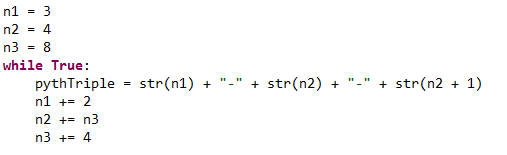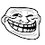# Is there a pattern in Pythagorean Triples?

Let's look at the first 5 Pythagorean Triples:

3-4-5, 5-12-13, 7-24-25, 9-40-41, 11-60-61

Are you noticing a pattern here?
The first number in each triple is just 3, 5, 7, 9, 11, adding 2 each time.
The second number in each triple is 4, then 12 (adding 8), then 24 (adding 12), then 40 (adding 16), then 60 (adding 20). You add 4 to the number you add to the number every time.
The third number in each triple is always just the second number + 1.
Here is a Python 3.4 algorithm to simulate this (sorry for bad quality).By this algorithm, the 1,000,000th Pythagorean triple is (drumroll please) 2,000,001-2,000,002,000,000-2,000,002,000,001!
Please let me know if you can find a proof for this algorithm.Note by Stefan Popescu
3 years, 2 months ago

This discussion board is a place to discuss our Daily Challenges and the math and science related to those challenges. Explanations are more than just a solution — they should explain the steps and thinking strategies that you used to obtain the solution. Comments should further the discussion of math and science.

When posting on Brilliant:

• Use the emojis to react to an explanation, whether you're congratulating a job well done , or just really confused .
• Ask specific questions about the challenge or the steps in somebody's explanation. Well-posed questions can add a lot to the discussion, but posting "I don't understand!" doesn't help anyone.
• Try to contribute something new to the discussion, whether it is an extension, generalization or other idea related to the challenge.

MarkdownAppears as
*italics* or _italics_ italics
**bold** or __bold__ bold
- bulleted- list
• bulleted
• list
1. numbered2. list
1. numbered
2. list
Note: you must add a full line of space before and after lists for them to show up correctly
paragraph 1paragraph 2

paragraph 1

paragraph 2

[example link](https://brilliant.org)example link
> This is a quote
This is a quote
    # I indented these lines
# 4 spaces, and now they show
# up as a code block.

print "hello world"
# I indented these lines
# 4 spaces, and now they show
# up as a code block.

print "hello world"
MathAppears as
Remember to wrap math in $$ ... $$ or $ ... $ to ensure proper formatting.
2 \times 3 $2 \times 3$
2^{34} $2^{34}$
a_{i-1} $a_{i-1}$
\frac{2}{3} $\frac{2}{3}$
\sqrt{2} $\sqrt{2}$
\sum_{i=1}^3 $\sum_{i=1}^3$
\sin \theta $\sin \theta$
\boxed{123} $\boxed{123}$

Sort by:

Look up Euclid's formula. In your case, you are increasing $m$ by a value of 1 at every step, and you set $n=1$.

- 3 years, 2 months ago

thank you!

- 3 years, 2 months ago